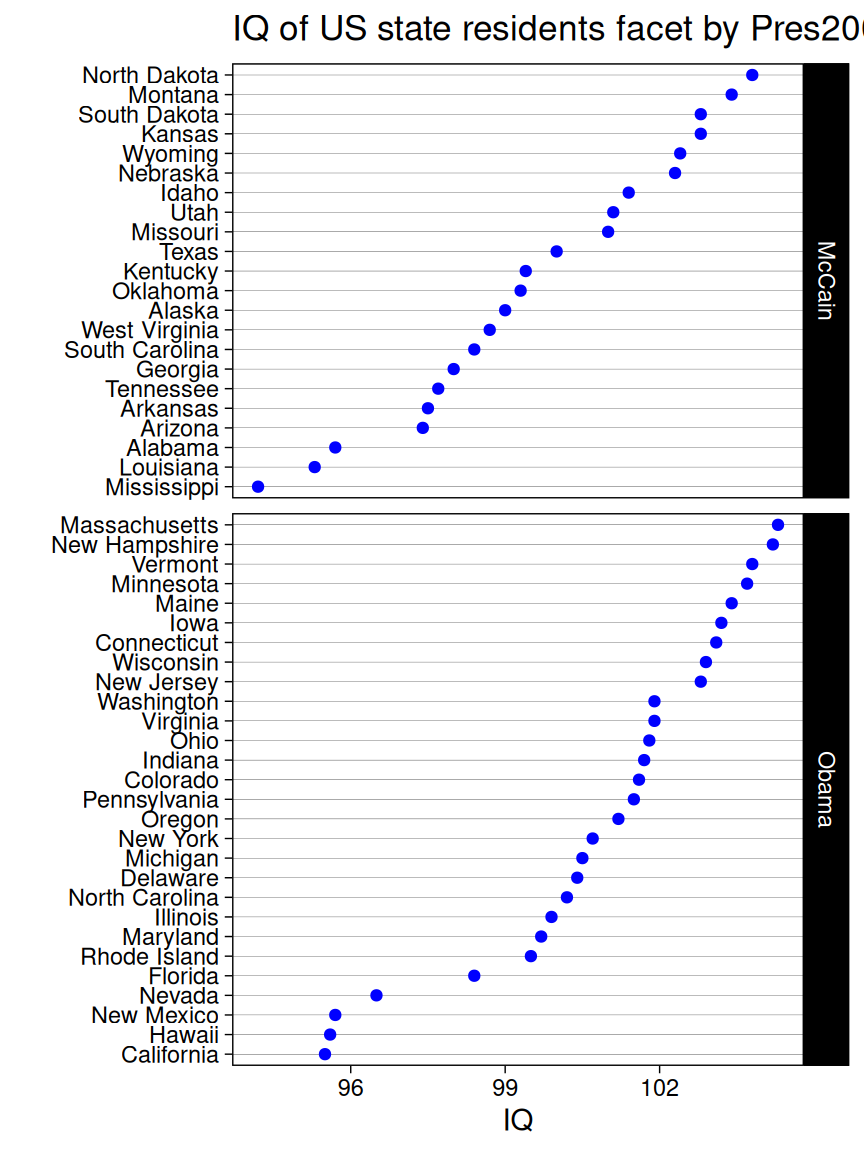# Chapter 10 Unidimensional categorical variables

In real-world datasets, categorical features are quite common but tricky during both the data pre-processing and visualization process. In this chapter, we will demonstrate several plotting options for the uni-dimensional categorical variables with `ggplot`.

## 10.1 Bar plot

There are two types of uni-dimensional categorical variables: nominal and ordinal. Here you will be shown how these variables should be plotted differently using bar plot under the same dataset.

### 10.1.1 Nominal data

Nominal data is data with no fixed category order and should be sorted from highest to lowest count (left to right, or top to bottom)

By default, R always sorts levels in alphabetical order. To reorder it by a sorted value, you can try `fct_reorder` , `fct_rev`, `fct_relevel` in the forcats package

``````library(vcdExtra)
library(ggplot2)
library(forcats)
library(dplyr)

Accident %>%
group_by(mode) %>%
summarise(freq = sum(Freq)) %>%
ggplot(aes(x=fct_reorder(mode,freq,.desc = TRUE),y=freq)) +
geom_bar(stat = "identity",fill = "cornflowerblue") +
ggtitle("Number of people with different modes in accident") +
xlab("") +
theme(panel.grid.major.x = element_blank())``````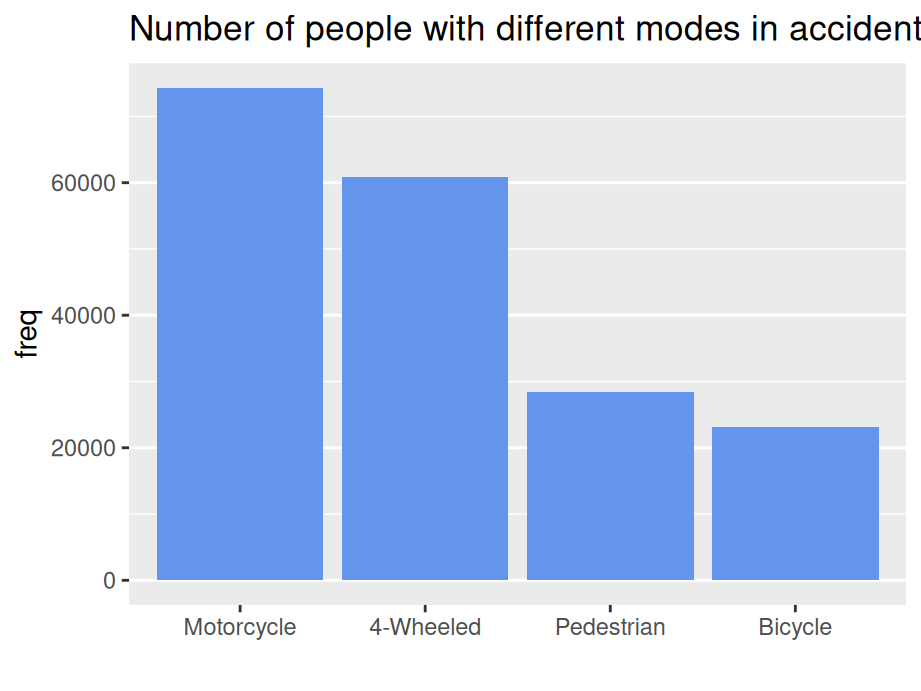… or top to bottom

``````Accident %>%
group_by(mode) %>%
summarise(freq = sum(Freq)) %>%
ggplot(aes(x=fct_rev(fct_reorder(mode,freq,.desc = TRUE)),y=freq)) +
geom_bar(stat = "identity",fill = "cornflowerblue") +
ggtitle("Number of people with different modes in accident") +
coord_flip() +
xlab("") +
theme(panel.grid.major.x = element_blank())``````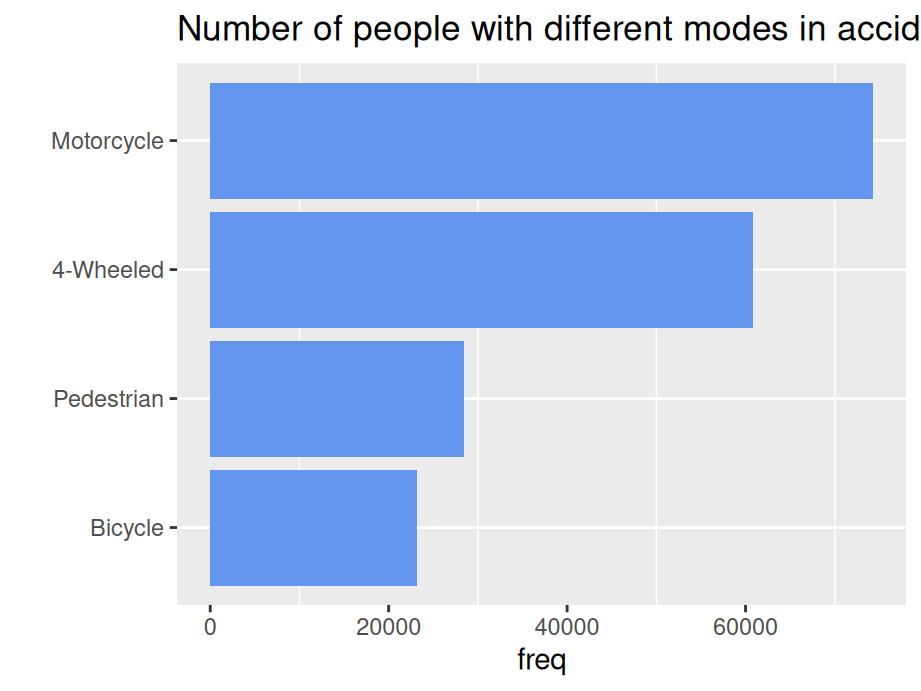### 10.1.2 Ordinal data

Ordinal data is data having a fixed category order and need to sort it in logical order of the categories (left to right)

``````Accident %>%
group_by(age) %>%
summarise(freq = sum(Freq)) %>%
ggplot(aes(x=age,y=freq)) +
geom_bar(stat = "identity",fill = "cornflowerblue") +
ggtitle("Number of people of different ages in accident") +
xlab("") +
theme(panel.grid.major.x = element_blank())``````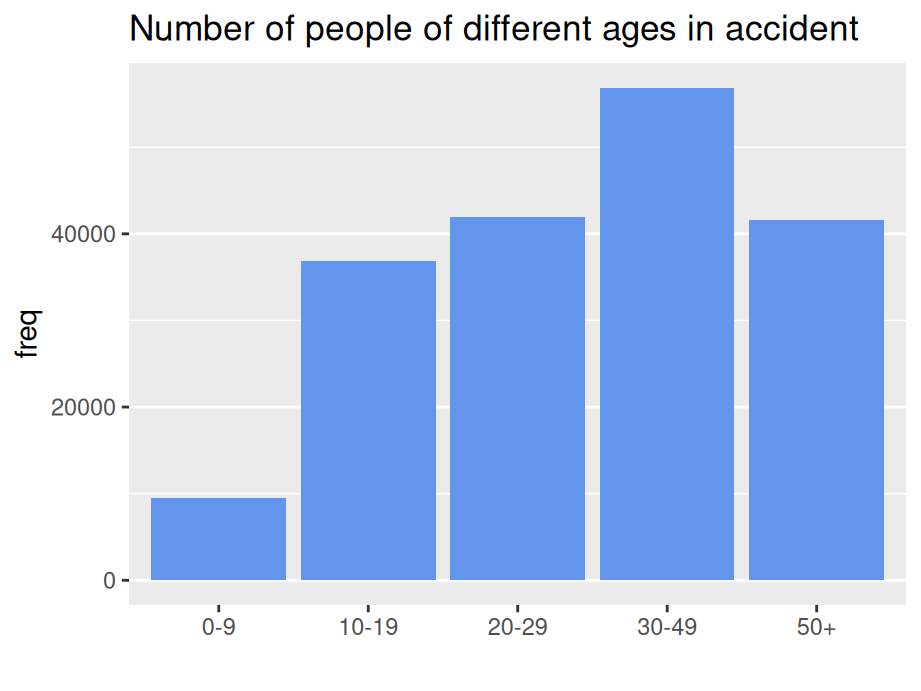Sort in logical order of the categories (starting at bottom OR top)

``````Accident %>%
group_by(age) %>%
summarise(freq = sum(Freq)) %>%
ggplot(aes(x=age,y=freq)) +
geom_bar(stat = "identity",fill = "cornflowerblue") +
ggtitle("Number of people of different ages in accident") +
xlab("") +
coord_flip() +
theme(panel.grid.major.x = element_blank())``````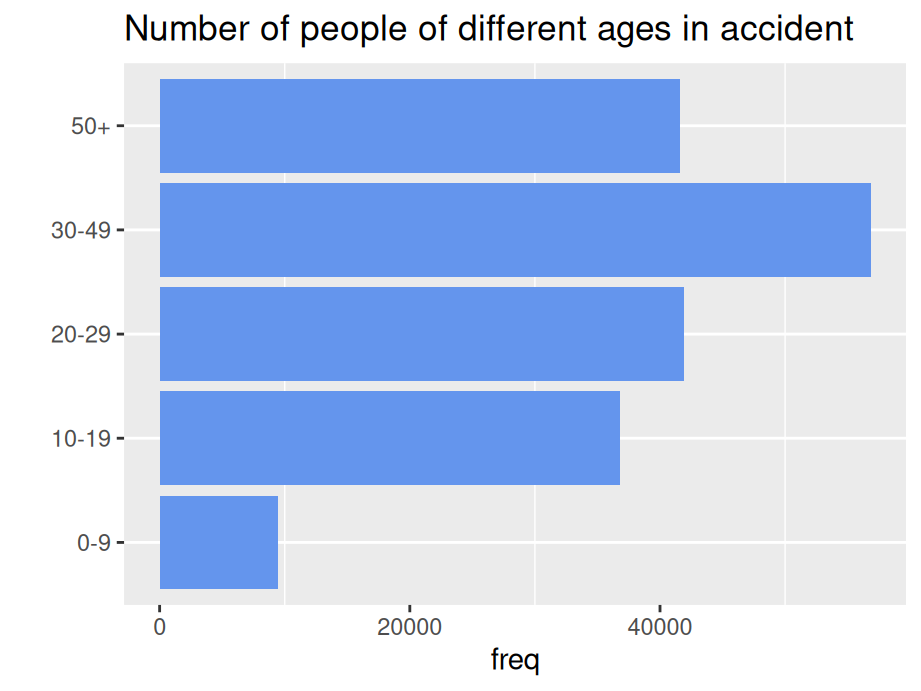## 10.2 Cleveland dot plot

Cleveland dot plot is a good alternative to bar plots, making plots more readable and comparable even with more data. Similarly, we also need to reorder the categorical variables just like what we’ve done for nominal bar plot.

``````library(Lock5withR)
ggplot(USStates, aes(x = IQ, y = fct_reorder(State, IQ))) +
geom_point(color = "blue") +
ggtitle("Avg. IQ for US states") +
ylab("") +
theme_linedraw()``````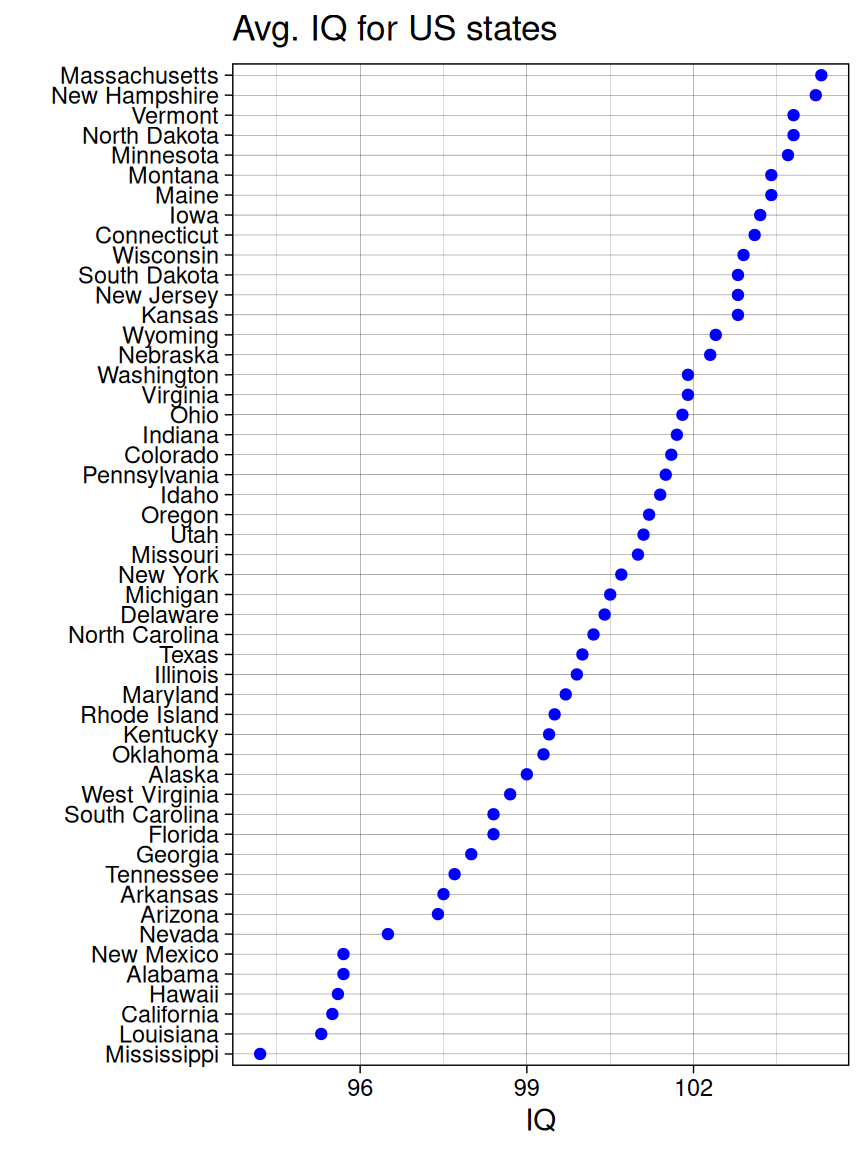### 10.2.1 Cleveland dot plot with multiple dots

Sort by Obese Rate

``````library(tidyr)
USStates %>%
select('State','Obese','HeavyDrinkers') %>%
gather(key='type',value='percentage',Obese,HeavyDrinkers) %>%
ggplot(aes(x=percentage, y=fct_reorder2(State,type=='Obese',percentage,.desc=FALSE), color = type)) +
geom_point() +
ggtitle("Obese rate & heavy drinker rate in US") +
ylab("") +
theme_linedraw()``````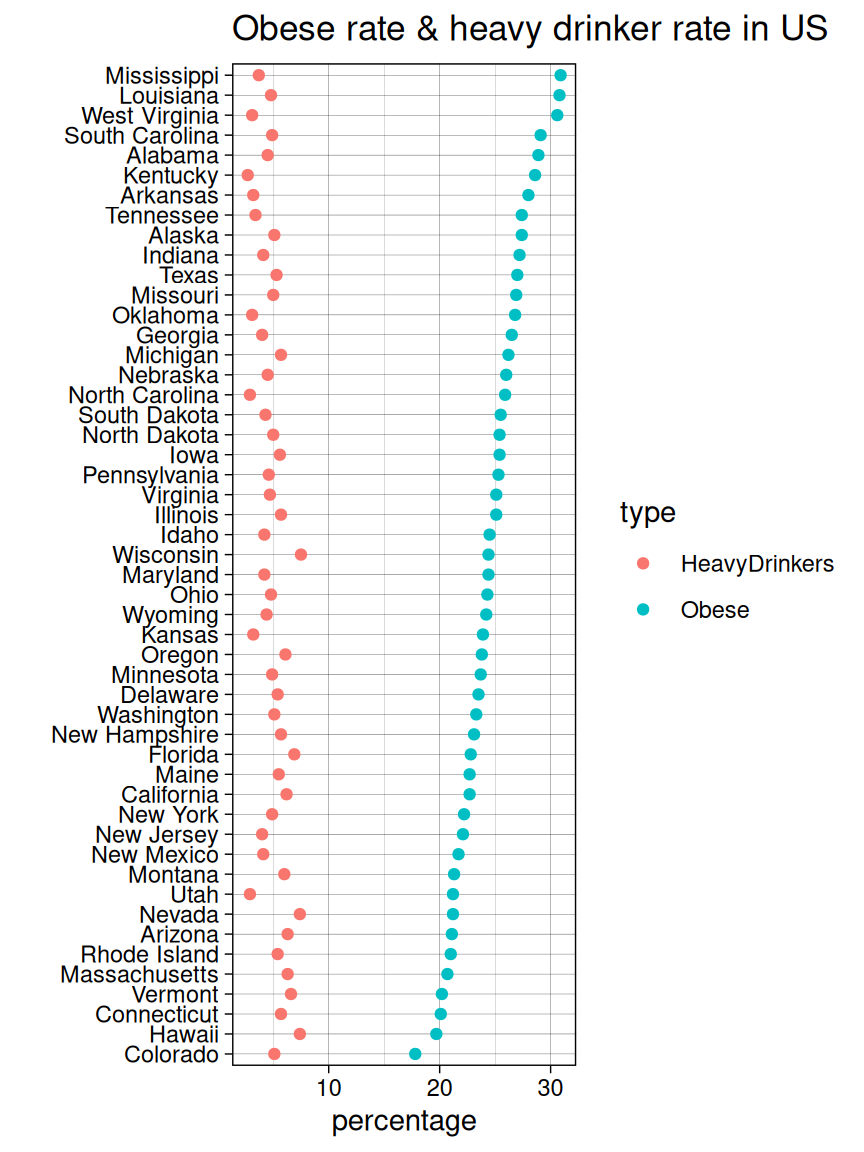### 10.2.2 Cleveland dot plot with facets

You can split the graph into small multiples using facet_grid().

``````ggplot(USStates, aes(x = IQ, y = reorder(State, IQ))) +
geom_point(color = "blue") +
facet_grid(Pres2008 ~ ., scales = "free_y", space = "free_y") +
ggtitle('IQ of US state residents facet by Pres2008') +
xlab("IQ") +
ylab('') +
theme_linedraw() +
theme(panel.grid.major.x = element_blank(), panel.grid.minor.x = element_blank())``````